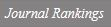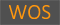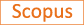Estimated Glomerular Filtration Rates Show Minor but Significant Differences Between the Single and Subgroup Creatinine-Based Chronic Kidney Disease Epidemiology Collaboration Equations

Title
Estimated Glomerular Filtration Rates Show Minor but Significant Differences Between the Single and Subgroup Creatinine-Based Chronic Kidney Disease Epidemiology Collaboration Equations
Authors
Park, SholhuiJeong, Tae-Dong
Ewha Authors
SCOPUS Author ID
정태동Issue Date
2019
Journal Title
ANNALS OF LABORATORY MEDICINE
ISSN
2234-38062234-3814Citation
ANNALS OF LABORATORY MEDICINE vol. 39, no. 2, pp. 205 - +
Keywords
Creatinine-based Chronic Kidney Disease Epidemiology Collaboration equationConstant modificationGlomerular filtration rate
Publisher
KOREAN SOC LABORATORY MEDICINE
Indexed
SCIE; SCOPUS; KCIDocument Type
Article
Abstract
The creatinine-based Chronic Kidney Disease Epidemiology Collaboration (CKD-EPI) equation can be calculated according to race, sex, and creatinine concentration (subgroup equation) or in the form expressed by one equation (single equation). Minor differences in the constants used in the CKD-EPI equations (subgroup vs single equations) could result in a significant difference in the estimated glomerular filtration rate (eGFR). We evaluated the impact of this difference in 79,709 Korean patients. The eGFR was calculated as an integer using the single and subgroup CKD-EPI equations. The differences in eGFR and GFR categories between the equations were analyzed. eGFR was higher in the subgroup equation than the single equation by 1 mL/min/1.73 m(2) for 12,476 (27.4%) Korean females. The GFR category based on the subgroup equation was reclassified using the single equation for 352 (0.77%) females. Based on the results, the constant of the single equation was optimized. There was no difference in eGFR values between equations using a multiplier of 1.0213 instead of 1.018 for the "white or other" females constant in the single CKD-EPI equation. Clinicians should carefully apply the CKD-EPI equation because eGFR values may differ by 1 mL/min/1.73 m(2) depending on the manner of calculation. To minimize these differences, the constants of the single equation should be revised.
DOI
10.3343/alm.2019.39.2.205
Appears in Collections:
의료원 > 의료원 > Journal papers
Files in This Item:
There are no files associated with this item.
Export
RIS (EndNote)
XLS (Excel)
XML